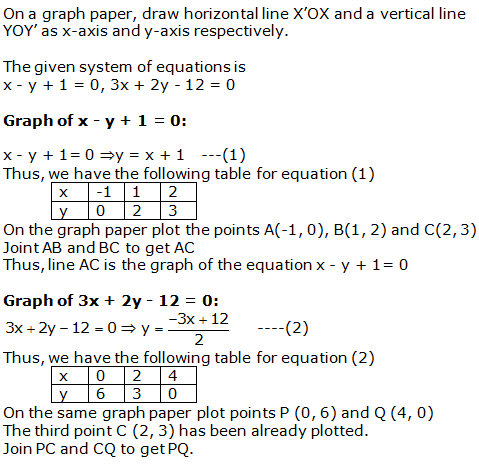# R S AGGARWAL AND V AGGARWAL Solutions for Class 10 Maths Chapter 3 - Linear equations in two variables

Page / Exercise

## Chapter 3 - Linear equations in two variables Ex. 3A

Solution 1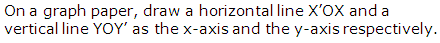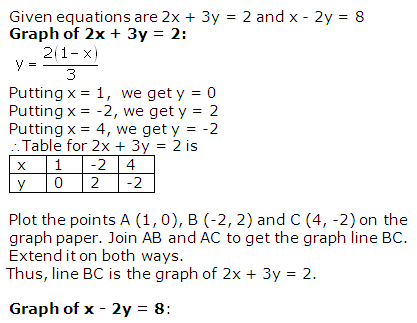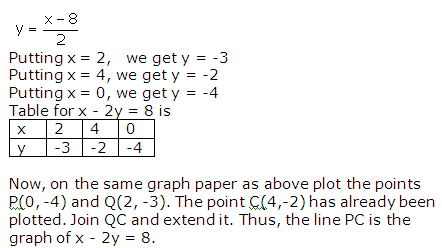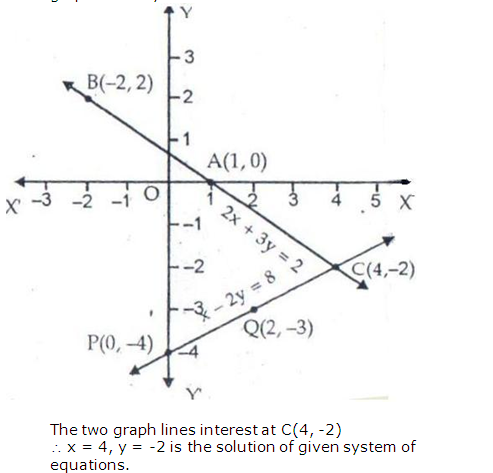Solution 3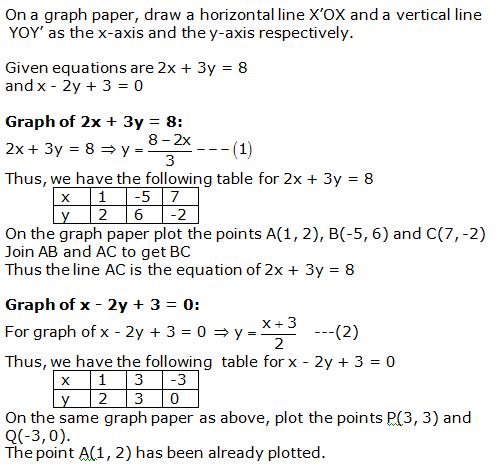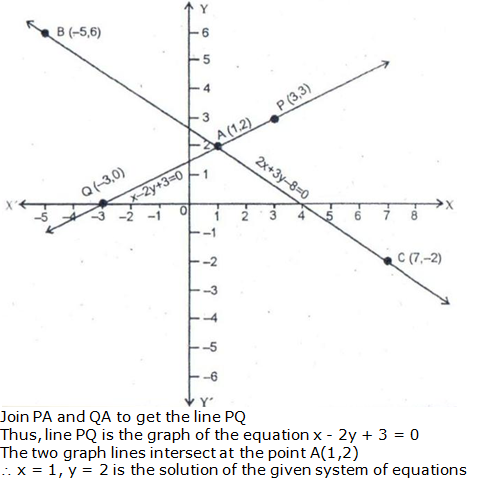Solution 4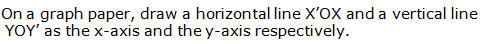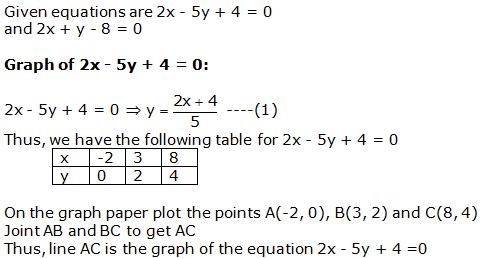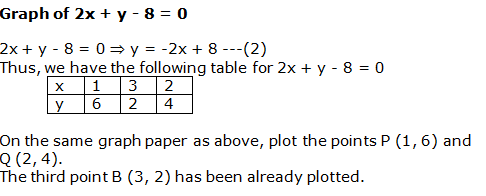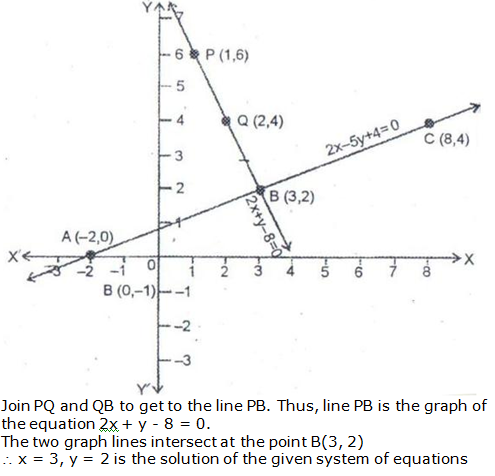Solution 5Since the two graphs intersect at (2, 3),

x = 2 and y = 3.

Solution 6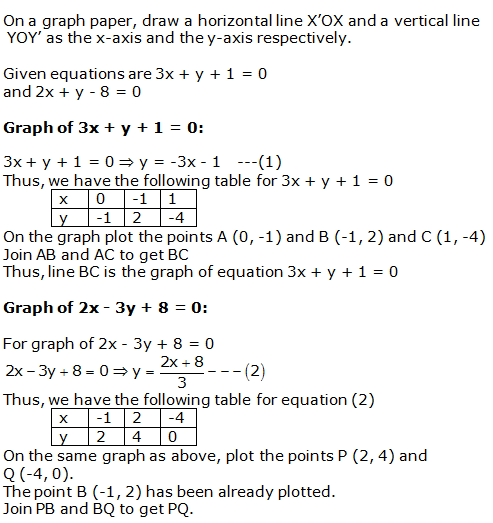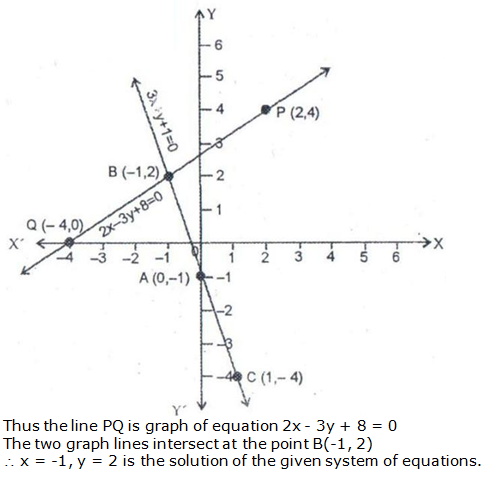Solution 7Since the two graphs intersect at (2, -3),

x = 2 and y = -3.

Solution 8Since the two graphs intersect at (-2, 3),

x = -2 and y = 3.

Solution 9Since the two graphs intersect at (-1, 2),

x = -1 and y = 2.

Solution 10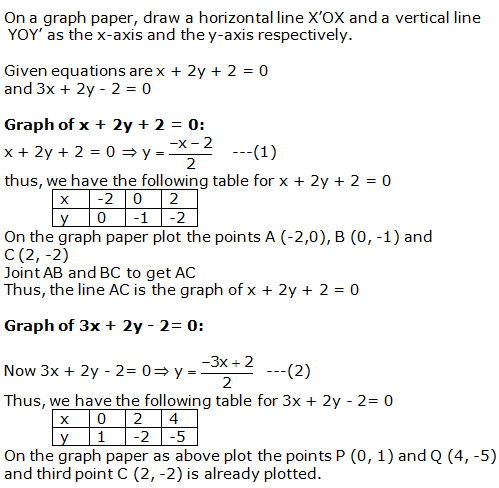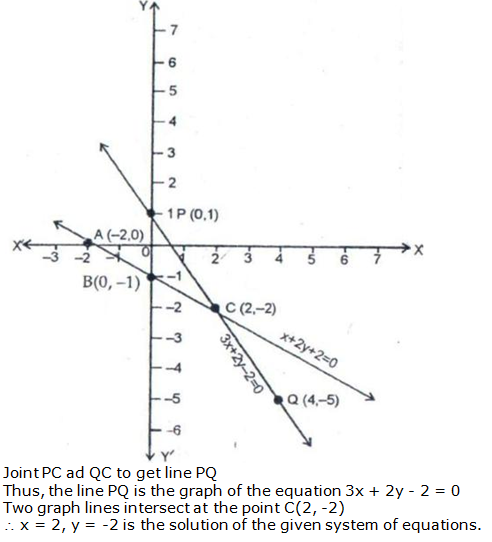Solution 11Solution 12Solution 13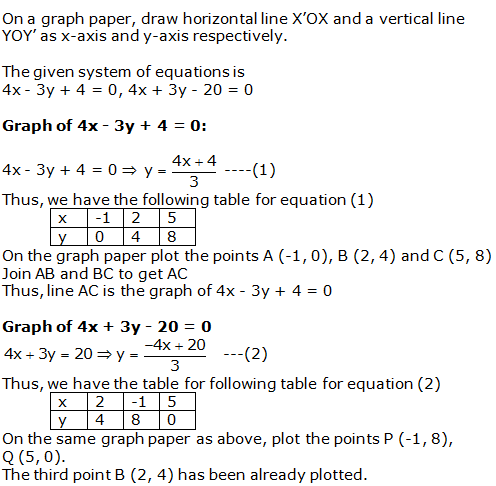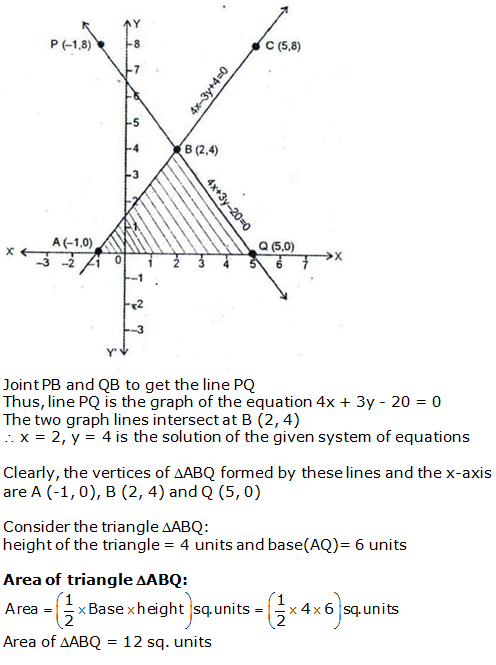Solution 14